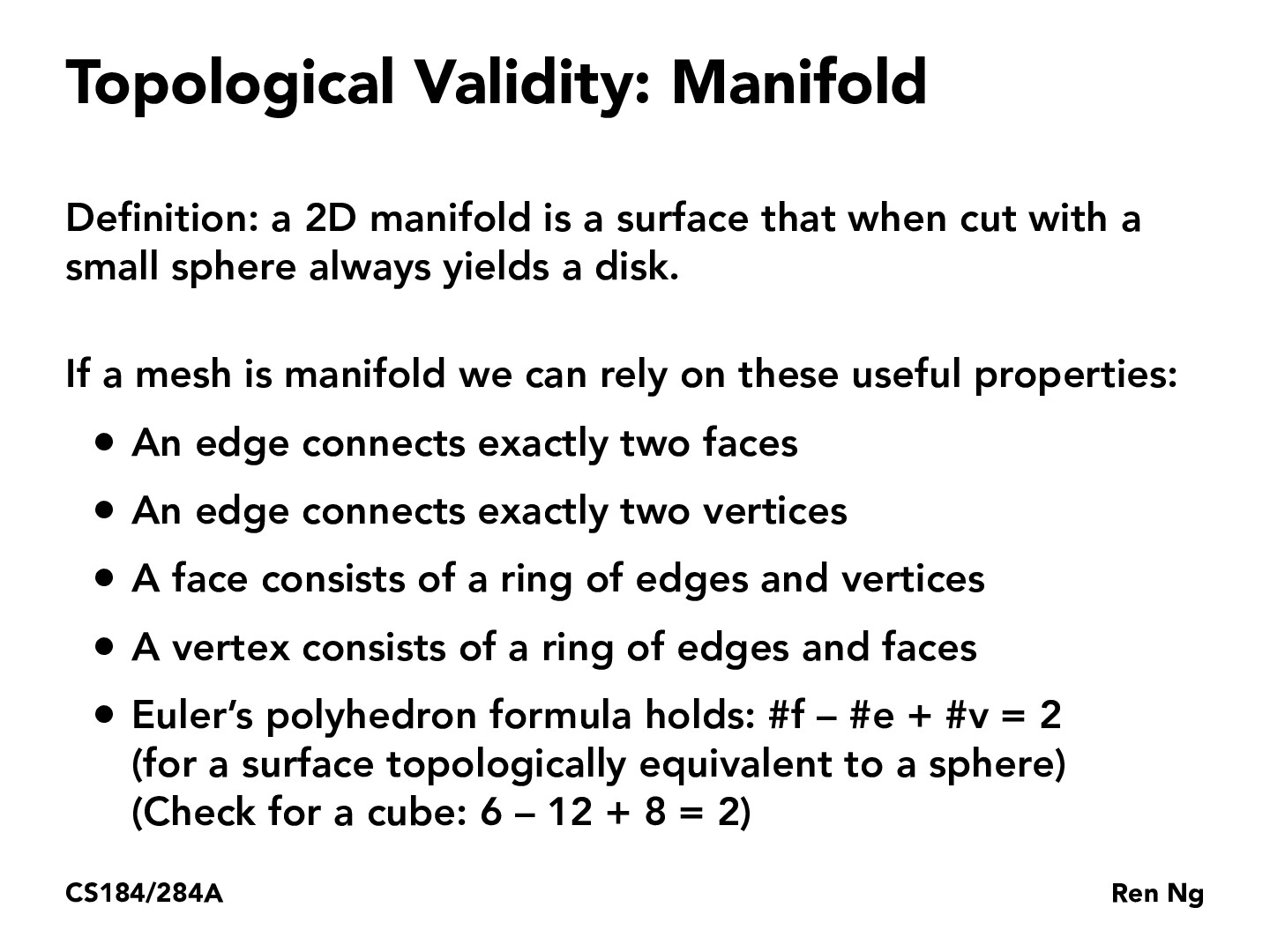Lecture 8: Meshes and Geometry Processing (18)caokevinc

If a surface is a 2D manifold then it satisfies Euler's polyhedron formula. Does this mean that all polyhedra are 2D manifolds? Prof Ren seems to add the last bullet as an interesting afterthought, but I wonder if the polyhedron formula can actually be useful in constructing meshes.hfan9

Wikipedia says that polyhedron are solids in 3D. Since manifolds are 2D, I don't think you can equate them. However, the last bullet notes that the formula holds when the surface (the 2D manifold) is topologically equivalent to a sphere, which I take to basically mean that the surface is closed (no boundaries, and it doesn't look like a ring). Then this manifold would be the surface area of a polyhedron, which is why the formula holds. The polyhedron itself is the 3D solid, but if you only looked at its 2D surface, you would get the manifold.

You must be enrolled in the course to comment